How Cheenta works to ensure student success?
Explore the Back-Story

# Graphing integer value function | Tomato Subjective 117This is a subjective problem from TOMATO based on Graphing integer value function.

Problem: Graphing integer value function

Let [x] denote the largest integer (positive, negative or zero) less than or equal to x. Let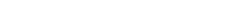be defined for all real numbers x.

(i) Sketch on plain paper, the graph of the function f(x) in the range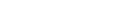(ii) Show that, any given real number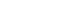, there is a real number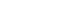such that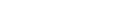Discussion:

First note that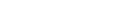is same as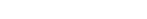.

It's graph between 0 to 1 looks like:Clearly [x] part only increments (or decrements) it by integer quantity as [x] is constant between any two integers. That for any integer k  for all ( x \in (k, k+1) ).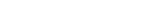,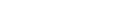. Hence graph of f(x) is as follows:Finally consider and arbitrary value. We take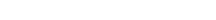. Then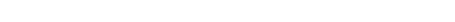(since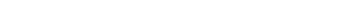)

## Chatuspathi:

• What is this topic: Graphing of functions
• What are some of the associated concept: Greatest Integer Function
• Where can learn these topics: Cheenta I.S.I. & C.M.I. course, discusses these topics in the ‘Calculus’ module.
• Book Suggestions: Play with Graphs, Arihant Publication

This is a subjective problem from TOMATO based on Graphing integer value function.

Problem: Graphing integer value function

Let [x] denote the largest integer (positive, negative or zero) less than or equal to x. Letbe defined for all real numbers x.

(i) Sketch on plain paper, the graph of the function f(x) in the range(ii) Show that, any given real number, there is a real numbersuch thatDiscussion:

First note thatis same as.

It's graph between 0 to 1 looks like:Clearly [x] part only increments (or decrements) it by integer quantity as [x] is constant between any two integers. That for any integer k  for all ( x \in (k, k+1) ).,. Hence graph of f(x) is as follows:Finally consider and arbitrary value. We take. Then(since)

## Chatuspathi:

• What is this topic: Graphing of functions
• What are some of the associated concept: Greatest Integer Function
• Where can learn these topics: Cheenta I.S.I. & C.M.I. course, discusses these topics in the ‘Calculus’ module.
• Book Suggestions: Play with Graphs, Arihant Publication

This site uses Akismet to reduce spam. Learn how your comment data is processed.

### Knowledge Partner